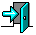# CS3013 Homework 4

Due date: Monday, October 13th

You must turn in your homework in written (by hand or typed) form by the beginning of class on the date it is due. Note, there are no late-dates allowed for this homework since a solution will be posted on the afternoon it is due to help with exam preparation.

The questions are based on material presented in class and reading material from the text.

Give brief answers to the following questions:

1. Briefly compare and contrast hard page faults with soft page faults.

2. Consider a demand-paging system with the page table stored in memory. The memory access time is 100 nanoseconds, then average soft page fault service time of 1 millisecond and the average hard page fault service time of 20 milliseconds. Currently, the system has a 30% page fault rate of which 70% are hard page faults. What is the effective memory access time?

3. Assume you have a page reference string for a process with 5 frames (initially all empty). The page reference string has length 13 with 7 distinct page numbers occurring in it. For any page replacement algorithm, what is the maximum number of page faults? What is the minimum number of page faults?

4. Consider the following page-reference string for one process:

```1,2,3,4,2,1,5,6,3,1,3,6,7,2,4,2,1,3,6
```
How many page faults would occur for the below replacement algorithms, assuming a total 4 frames available for the process. Assume pure demand paging (all frames are initially empty), so the first page faults will all cost one fault each.

• First In First Out (FIFO) replacement
• Least Recently Used (LRU) replacement
• Optimal replacement
5. Consider the following page-reference string for one process:

```1,2,3,4,2,1,5,6,3,1,3,6,7,2,4,2,1,3,6
|   |
X   Y
```
Assume past window interval of 5 page references.

• What are the working sets for the process at time X and at time Y?
• Assuming a total 4 frames available for the process, would it be thrashing at time X? How about at time Y?
• What is the minimum number of frames needed be assigned to the process to avoid thrashing?
6. Software written to control hardware generally has two parts to it: a device dependent layer and a device independent layer. What are the advantages of such an approach? Enumerate the functions of each layer.

7. How is a block device is different from a character device? Give at least one example for each I/O device type.

8. Consider the following file distribution on a disk cylinder:

```Cylinder:  1, 2, 3, 4, 5, 6, 7, 8, 9,10,11,12,13,14,15,16,17,18
Files:     x     x        x              x  x        x     x  x
Req order: 3     6        1              4  2        8     5  7
```
The time to move the disk arm between each cylinder is 1ms and the disk arm is currently located at cylinder 13 (and was moving up). Assuming that all the read requests are already made prior to the disk scheduling, calculate the average seek times for the following arm scheduling algorithms:

1. FCFS scheduling
2. SSF scheduling
3. Elevator (SCAN) schedulingReturn to the CS3013 Home Page

Send all questions to the TA mailing list.